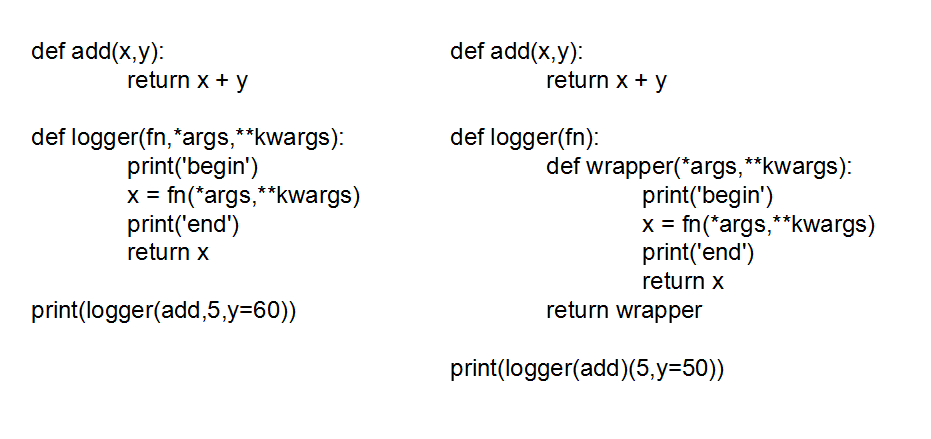2018-09-2700

## 目录

1. 什么是装饰器
2.举个栗子
1.引子
2.提取参数
3.柯里化
4.装饰器语法糖
3.复杂的栗子
4.带参装饰器
1. 文档字符串
2. 前面装饰器的副作用
3. 解决方案一
4. 解决方案二

### 2.举个栗子

#### 1.引子

``````#功能函数
return x+y

#装饰函数
def logger(fn):
print('frist')
x = fn(4,5)
print('second')
return x

#print('frist')
#print('secend')
#  x = fn(4,5)  ==> x = 4 y= 5  x= 4+5 = 9
#return 9

``````
``````frist
second
9
``````

#### 2.提取参数

x,y的参数都放在logger函数内部了，影响函数的灵活性，此处我们可以提取出来。

``````def add(x,y):
return x + y

def logger(fn,*args,**kwargs):
print('frist')
x = fn(*args,**kwargs)
print('second')
return x

``````
``````frist
second
12
``````

#### 3.柯里化

``````def add(x,y):
return x + y
def logger(fn):
def wrapper(*args,**kwargs):
print('begin')
x = fn(*args,**kwargs)
print('end')
return x
return wrapper

``````
``````begin
end
16
`````````bash```def add(x,y):
return x + y

def logger(fn,*args,**kwargs):        def logger(fn):  #参数剥离
def newfunction(*args,**kwargs):  #新定义一个函数，logger函数返回也是这个函数名字
print('frist')                           print('frist')
x = fn(*args,**kwargs)  == >             x = fn(*args,**kwargs)
print('second')                          print('second')
return x                               return x
return newfunction

``````

``````def add(x,y):
return x + y

def logger(fn):  #参数剥离
def newfunction(*args,**kwargs):  #新定义一个函数，logger函数返回也是这个函数名字

print('frist')
x = fn(*args,**kwargs)
print('second')
return x

return newfunction

``````
``````frist
second
16
``````

#### 4.装饰器语法糖

``````#再次变形。。。
return x + y

def logger(fn):
def wrapper(*args,**kwargs):
print('begin')
x = fn(*args,**kwargs)
print('end')
return x
return wrapper

##调用方法1：

##调用方法2：

##调用方法3： python给我们的语法糖

return x + y

``````
``````begin
end
1112
begin
end
14
begin
end
85
``````

### 3.复杂的栗子

``````import datetime
import time

def logger(fn):
def warp(*arges,**kwarges):
print("arges={},kwarges={}".format(arges,kwarges))  #打印函数的两个参数
start = datetime.datetime.now()  #获取函数运行的开始时间

duratime = datetime.datetime.now() - start  #获得函数的运行时间
print("function {} took {}s".format(fn.__name__,duratime.total_seconds()))  #打印函数的运行时间

return ret   #返回fn的结果 ，fn = x+y ==> 返回x+y的值。  x = 4 y= 11 ==> return 11
return warp  #返回warp的 return ==> ret 的return ==> return 11 函数的最终结果为11

@logger
print("oooooook")
time.sleep(1.5)
return x+y

#如果充分理解了每个小部件，这个简单的完整版本也是很好理解的了。
#1，logger是个装饰器，而且使用了柯里化技术
#
#
``````
``````arges=(4,),kwarges={'y': 11}
oooooook
15
``````

``````import datetime
import time

#####################################装饰开始############################################
def logger(fn):  #拿到函数名称
def warp(*arges,**kwarges):  #拿到函数带过来的参数开始装饰
print("arges={},kwarges={}".format(arges,kwarges))   #来试试打印两个参数
start = datetime.datetime.now()  #

duratime = datetime.datetime.now() - start  #
print("function {} took {}s".format(fn.__name__,duratime.total_seconds()))

return warp  # logger的返回结果是warp，warp的返回值是ret ，ret的返回值是 add函数的执行结果（原函数的功能完整的保留了）

#####################################装饰完成############################################

@logger  #装饰工厂
print("oooooook")
time.sleep(1.5)
return x+y

``````
``````arges=(4,),kwarges={'y': 11}
oooooook
15
``````

### 4.带参装饰器

#### 1. 文档字符串

``````def add(x,y):
"""This is a function of addition"""
a = x+y
return x + y

``````
``````function name is add
function doc = This is a function of addition

Help on function add in module __main__:

This is a function of addition

None
``````

#### 2. 前面装饰器的副作用

``````def add(x,y):
return x + y

def logger(fn):
"This is logger doc"
def wrapper(*args,**kwargs):
"This is wrapper doc"
print('begin')
x = fn(*args,**kwargs)
print('end')
return x
return wrapper

return x + y

``````
``````begin
name = wrapper
doc = This is wrapper doc
end
85
``````

#### 3. 解决方案一

1. copy原函数的属性 copy_properties
2. 装饰器 logger
``````def copy_properties(src, dst): # 把src的相关属性赋值给dst  (fn,wrap)
dst.__name__ = src.__name__
dst.__doc__ = src.__doc__

def logger(fn):
"""'This is a function of logger'"""
def wrap(*arges,**kwarges): #
"""'This is a function of wrap'"""
print('<>-<>-<>-<>-<>-<>-<>-<>-<>-<>-<>-<>-<>-<>-<>-<>-<>-<>')
x = fn(*arges,**kwarges)
print('<>-<>-<>-<>-<>-<>-<>-<>-<>-<>-<>-<>-<>-<>-<>-<>-<>-<>')
return x
copy_properties(fn,wrap)    #思考1：为什么放在这个位置调用
return wrap

@logger
"""'This is a function of add'"""
return x+y

``````
``````<>-<>-<>-<>-<>-<>-<>-<>-<>-<>-<>-<>-<>-<>-<>-<>-<>-<>
doc='This is a function of add'
<>-<>-<>-<>-<>-<>-<>-<>-<>-<>-<>-<>-<>-<>-<>-<>-<>-<>
10
``````

#### 4. 解决方案二

1. copy原函数的装饰器 copy_properties1
2. 装饰器 logger
``````def copy_properties(src, dst): # 把src的相关属性赋值给dst  (fn,wrap)
dst.__name__ = src.__name__
dst.__doc__ = src.__doc__

#利用前面的知识我们可以对copy_properties轻松进行变形
def copy_properties1(src): #  把src的相关属性赋值给dst  (fn,wrap)
def _copy(dst):
dst.__name__ = src.__name__
dst.__doc__ = src.__doc__
return dst
return _copy
``````

``````def logger(fn):
"""'This is a function of logger'"""
@copy_properties1(fn) #wrap = copy_properties(fn)(wrap)
#== > 柯里化 两次传入参数 src = fn ， dst = wrap 新的wrap函数的属性已经替换为原函数的。

def wrap(*arges,**kwarges): #wrap = copy_properties(fn)(wrap)(*arges,**kwarges)
"""'This is a function of wrap'"""
print('>->->->->->->->->->->->->->->->->->->->->->->->->->')
x = fn(*arges,**kwarges)
print('<-<-<-<-<-<-<-<-<-<-<-<-<-<-<-<-<-<-<-<-<-<-<-<-<-<')
return x

return wrap

"""'This is a function of add'"""
return x+y

``````>->->->->->->->->->->->->->->->->->->->->->->->->->